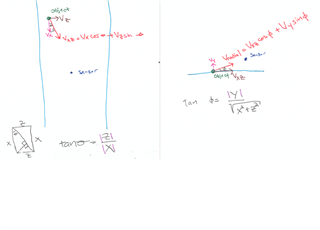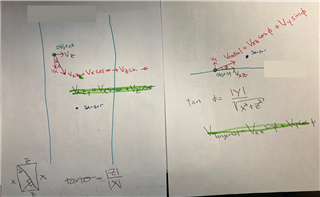If you have a related question, please click the "Ask a related question" button in the top right corner. The newly created question will be automatically linked to this question.

# AWR1843AOP: AWR1843AOP Radial vs Linear / Real Velocity

Part Number: AWR1843AOP

Hello,

I took a stab at deriving X, Y, Z velocity from the radial velocity reported by the chip.

Can you look over my math / trigonometry / equations / vector break down and let me know if my theory is correct?

Thank you

Chris• Also just to confirm, positive radial velocity reported from the chip is when the object is moving towards the sensor?

• Hi Chris,

The equations look correct to me provided the x, y and z directions are assumed properly.

I think the radial velocity reported by chip is positive as object moves away from radar since phase change will then only be positive. Please refer to slide 37 of this pdf: https://training.ti.com/sites/default/files/docs/mmwaveSensing-FMCW-offlineviewing_0.pdf . Here it clearly shows the phasor moves in anti-clockwise motion (phase is positive) only if object moves away from radar and vice-versa.

Let me know if you have any questions.

Thanks and Regards,

Akshay.

• I wanted to ask about a special situation regarding the first drawing on the left where Vxz=Vx cosΘ + Vz cosΘ

if z=0 then Θ = 0 and Vxz = Vx  since cos(0)=1 and sin(0)=0 | it doesnt matter what Vz is as it is not measured/reported in the radial velocity

is that correct?

if so then if x=0, Vxz=Vz and if x and z=0 then the object would be at the same position as the sensor and any velocity would not be radial so Vxz=0

is that correct?

• Hi Chris,

Give me some time. Let me go through this once more and get back.

Thanks and Regards,

Akshay.

• I thought more and wanted to add.

I believe the special case assessment is still correct but to add, if z=0 then Vz can not be determined as it is purely tangential and not measured/reported by the chip.

and if z=x=0, Vz and Vx are purely tangential and neither can be determined.

• I added the tangential component to my analysis• Hi Chris,

From your diagram analysis you are right. If x=0, z=0, sensor and object are in same position. While if z = 0, Vz is not determined and is purely tangential, so not measured by the chip.

Thanks and Regards,

Akshay.